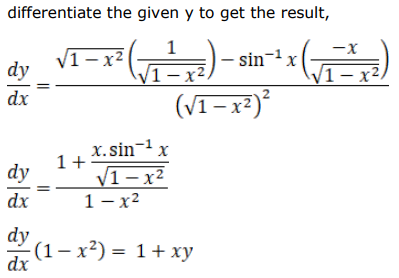# Solve this followingQuestion:

If $y=\frac{\sin ^{-1} x}{\sqrt{1-x^{2}}}$, prove that $\left(1-x^{2}\right) \frac{d y}{d x}=(x y+1)$

Solution: